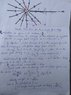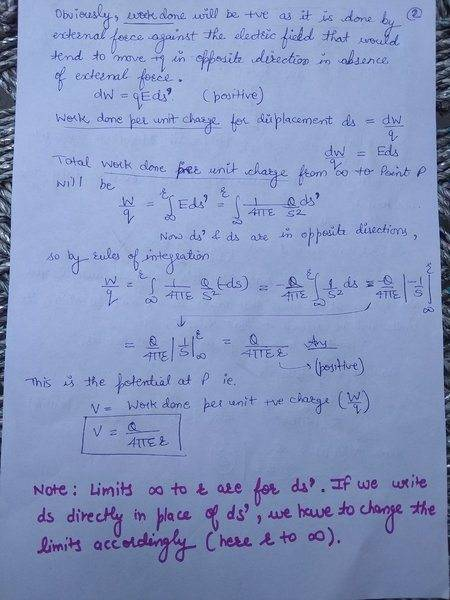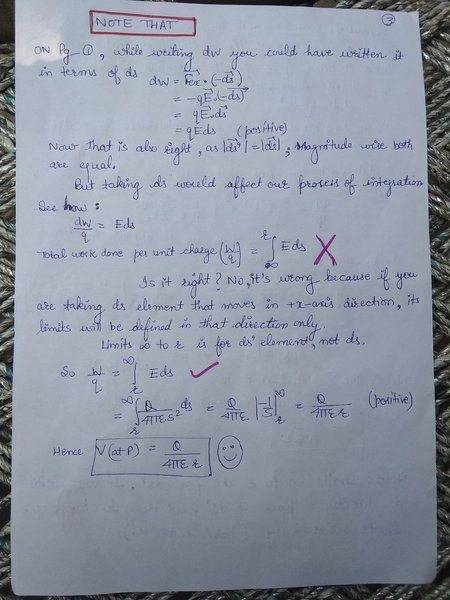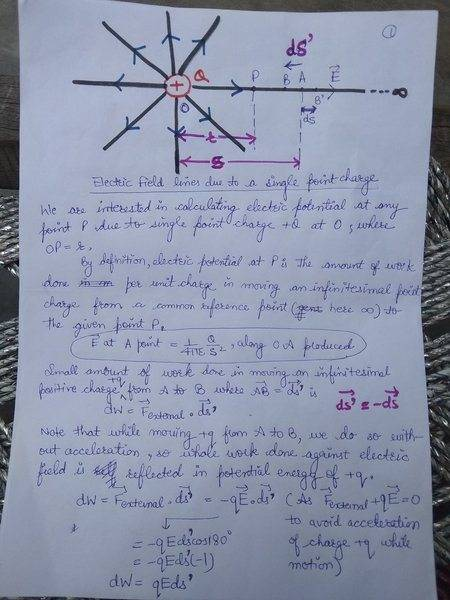# Electric Potential due to a single point charge

Sunanda
Please refer to the image attached. So, my doubt is:

While calculating dW in the derivation, we know this work is being done by external force, because only then the unit positive charge can be made to move towards the charge +Q. So dW should be equal to Fext.dx but here in the book it is shown equal to E.dx
Isn't it?

Remarks: Here above, bold letters represent vector form.

#### Attachments

•IMG_20180405_134300.jpg
41.5 KB · Views: 804
Last edited:

Mentor
There is a bit of cheating here. ##\mathbf{E} = \mathbf{F}/q##, so for a unit charge, ##\mathbf{E}## and ##\mathbf{F}## will give the same numerical value. You can check that the units you get for ##W## are not correct (C/F instead of C2/F = J).

Sunanda
There is a bit of cheating here. ##\mathbf{E} = \mathbf{F}/q##, so for a unit charge, ##\mathbf{E}## and ##\mathbf{F}## will give the same numerical value. You can check that the units you get for ##W## are not correct (C/F instead of C2/F = J).
But it's not numerical value that I'm concerned about. I know that numerically both F and E are equal to each other, for unit positive charge.
My doubt is related to direction. Fext has direction opposite to that of E. While the former is directed towards the source charge, the latter is directed away from it. Will you please answer now? How is this right?

willem2
My doubt is related to direction. Fext has direction opposite to that of E. While the former is directed towards the source charge, the latter is directed away from it. Will you please answer now? How is this right?
The definition of potential from your notes is rather imprecise.
The amount of work done in carrying a unit of positive charge from ∞ to P.
The electrical forces will do negative work on P while it moves, and what is wanted is is the positive work, needed to cancel this so the particle ends up with the same kinetic energy as it started with.Your notes simply calculate the work done by the electric field and then get the sign wrong. dW = E.dr should come out negative as E has the opposite direction as dr. It really makes no sense to consider the magnitude of the external force however. The particle could just as well be acceleratied at some point far away, and then be shot towards the point P

Let'sthink
I agree with Willem2, but I have some problems with the quoted definition; "The amount of work done in carrying a unit of positive charge from ∞ to P." . It should be work done per unit charge in carrying a very small almost infinetesimal (test charge) amount of charge ... " This is more correct as such and also it makes the definition dimension-wise correct. Note dimensions of potential and work are not identical. Similar correction has to be done in giving the definition of electric field.

Last edited:
•Sunanda
Sunanda
The definition of potential from your notes is rather imprecise.

The electrical forces will do negative work on P while it moves, and what is wanted is is the positive work, needed to cancel this so the particle ends up with the same kinetic energy as it started with.Your notes simply calculate the work done by the electric field and then get the sign wrong. dW = E.dr should come out negative as E has the opposite direction as dr. It really makes no sense to consider the magnitude of the external force however. The particle could just as well be acceleratied at some point far away, and then be shot towards the point P

Dear Willem2,
I guess you missed the first line of derivation in which I have mentioned this whole derivation is from the textbook. I was having problem in grasping the book's derivation, so I posted it as a question here. They are clearly not my notes.

Secondly, you have added a term dr. Can I know where is it directed to- along the electric field or opposite to it? Perhaps then only I might be able to fullyunderstand your point of view.

Sunanda
I agree with Willem2, but I have some problems with the quoted definition; "The amount of work done in carrying a unit of positive charge from ∞ to P." . It should be work done per unit charge in carrying a very small almost infinetesimal (test charge) amount of charge ... " This more correct as such and also it makes the definition dimension-wise correct. Note dimensions of potential and work are not identical. Similar correction has to be done in giving the definition of electric field.
Yes, you are right about the definition. It needed correction. But this whole derivation that I have jotted down on paper, is from my textbook. I have mentioned this immediately below the figure. Though my doubt is yet to be cleared, thankyou for the correction.

Let'sthink
Yes, you are right about the definition. It needed correction. But this whole derivation that I have jotted down on paper, is from my textbook. I have mentioned this immediately below the figure. Though my doubt is yet to be cleared, thankyou for the correction.
We have to be careful here. Either you work with positive values such as E and dr or you work with vectors: E and dr.
First using vectors: Consider any r' between r and ∞. r' is known as dummy variable which will occur under integral sign[Normally textbooks do not distinguish in this way and think that students will understand automatically that what is under Integral sign or even in the expression of dw is dummy variable
First consider dw for E pointing away from given charge and dr' also pointing away from given charge then
dw = E.dr' = Edr', where E and dr' are magnitudes and hence are positive but what this work will do take charge towards the charge away ? or nearer answer is away but we wish to bring it closer therefore for our consideration E and dr' are not pointing in same direction but in opposite direction and hence
dw = E.dr' = -Edr', where E and dr' are magnitudes and hence are positive. This truly represents the work don by E and is therefore negative but the work done by the external agency will be positive will be proved so when you integrate the expression. according to rules of mathematics after integration you would put the value of r' as r and then subtract putting r' = ∞.

If you do not use vector notation then it is obvious work done by E if you tend to increase is Edr' and if you wish to decrease it is -Edr'

All said and done you are finding the work done by the electric field if electric field does he work it will be positive and the test charge will gain energy but we want to take the charge there without increasing its energy hence potential energy will be reduced. Reverse will happen when we bring it closer from ∞.to r we will do work (equivalently means electric field does negative work) and that energy spent by us will be stored as potential energy of the moved charge.

Last edited:
Let'sthink
There are two types of definitions of physical variable/concepts. One is theoretical, which relates the new concept with the earlier ones and other is operational which states how it can be measured. Some are in between which creates confusion. It is theoretical but is worded in an experimental garb. Basically
E = -dV/dr or dV = -Edr or E = -grad V or E.dr = -dV = -[(∂V/∂x)dx + (∂V/∂y)dy + (∂V/∂z)dz]
These definitions do not need the concept of test charge and states that the space around a point charge acquires a scalar property at every pint and a related vector property at every point in the neighborhood.

Sunanda
"First consider dw for E pointing away from given charge and dr' also pointing away from given charge then
dw = E.dr' = Edr', where E and dr' are magnitudes and hence are positive but what this work will do take charge towards the charge away ? or nearer answer is away but we wish to bring it closer therefore for our consideration E and dr' are not pointing in same direction but in opposite direction and hence
dw = E.dr' = -Edr', where E and dr' are magnitudes and hence are positive. This truly represents the work done by E and is therefore negative but the work done by the external agency will be positive will be proved so when you integrate the expression. according to rules of mathematics after integration you would put the value of r' as r and then subtract putting r' = ∞.

I understood everything you wrote, except the few lines in this paragraph. Hope you will explain that too. So, I proceed:

You wrote:
but we wish to bring it closer therefore for our consideration E and dr' are not pointing in same direction but in opposite direction and hence
dw = E.dr' = -Edr', where E and dr' are magnitudes and hence are positive.
I get that E and dr' are not pointing in the same direction as now dr' is directed towards the source charge placed at origin. That is, it points towards the negative x-axis. But I did it as:

Small amount of work done per unit charge in bringing a test charge from infinity to the point P at a distance r from the source charge +Q = dW. Now this dW has to done by the external force, say F. And F should be such that F + qE = 0. Therefore, F = -qE.

So dW= F.dr' = -qE.dr' = -q(E dr' cos 180°)
= -(-E dr') = E dr'

Is it wrong? Why?

Let'sthink
I understood everything you wrote, except the few lines in this paragraph. Hope you will explain that too. So, I proceed:

You wrote:

I get that E and dr' are not pointing in the same direction as now dr' is directed towards the source charge placed at origin. That is, it points towards the negative x-axis. But I did it as:

Small amount of work done per unit charge in bringing a test charge from infinity to the point P at a distance r from the source charge +Q = dW. Now this dW has to done by the external force, say F. And F should be such that F + qE = 0. Therefore, F = -qE.

So dW= F.dr' = -qE.dr' = -q(E dr' cos 180°)
= -(-E dr') = E dr'

Is it wrong? Why?
E dr' is wrong because it means as r' is decreased by dr' which will be eventually negative the energy will decrease but as we do work on the test charge energy should increase. Compare with V = mgh as h increases pe increases, in this case as r' decreases pe should increase. We want to write -Edr' so that when we put dr' as <0 -Edr' becomes >0.

•Sunanda
Let'sthink
There are two types of definitions of physical variable/concepts. One is theoretical, which relates the new concept with the earlier ones and other is operational which states how it can be measured. Some are in between which creates confusion. It is theoretical but is worded in an experimental garb. Basically
E = -dV/dr or dV = -Edr or E = -grad V or E.dr = -dV = -[(∂V/∂x)dx + (∂V/∂y)dy + (∂V/∂z)dz]
These definitions do not need the concept of test charge and states that the space around a point charge acquires a scalar property at every pint and a related vector property at every point in the neighborhood.

What is your take on this Ms Sunanda?

Sunanda
E dr' is wrong because it means as r' is decreased by dr' which will be eventually negative the energy will decrease but as we do work on the test charge energy should increase. Compare with V = mgh as h increases pe increases, in this case as r' decreases pe should increase. We want to write -Edr' so that when we put dr' as <0 -Edr' becomes >0.

I have taken dr' to be the magnitude of vector dr' , which is now pointing in the negative x-axis. In that sense, Edr' which I found out in the final result will be positive. I understand that work done by external agent to move a positive charge towards positive source charge will be positive. But if I integrate this dW =Edr' (which at this stage is positive) between limits infinity to r, total work done in the process i.e. W comes out to be negative. Perhaps that is because we integrated from infinity to r, rather than r to infinity. In mathematics,
Integral of a quantity between limits a and b= -(Integral of the same quantity between limits b and a)

Now as per the textbooks and derivation present everywhere, they have found out dW to be negative, equal to -E dr' which upon integration from limits infinity to r gives the total value as positive. This is desirable logically. Now your -E dr' is positive, my Edr' is also positive assuming dr' represents only magnitude of decrement. But the point is if it comes out positive, total work done will be negative. And that can't be true. Kindly explain how Edr' (or in your case -Edr') comes out negative mathematically?

Thankyou.

Sunanda
If I compare it with V= mgh, or gravity. If I carry book up from the floor against the gravitational force, the work done by me on the book will be positive. Because both the force and displacement will be in same direction. Right? Here also, while writing dW= F.dr' I assumed my infinitesimal work dW should have come positive because force and displacement are in the same direction. But it is not so in the derivations present everywhere. Their dW is negative which upon integration from infinity to r yields positive work done. How dW is negative? Mathematically, isn't dW= F.dr' ?

Note: (dr' = -dr')

Sunanda
Upon re-reading you explanations, including explanations on other threads, finally my most of the doubts have got cleared including the ones mentioned in the previous two posts. So you needn't clarify them now ☺️. Thankyou for your time :) But there's just one doubt left related to this thread that I will post soon, I request you to answer that too. I shall be really grateful. :)

Let'sthink
Upon re-reading you explanations, including explanations on other threads, finally my most of the doubts have got cleared including the ones mentioned in the previous two posts. So you needn't clarify them now ☺️. Thankyou for your time :) But there's just one doubt left related to this thread that I will post soon, I request you to answer that too. I shall be really grateful. :)
You are welcome. Although you have understood still I repeat positive or negative work done is concluded by whether we support or oppose the existing interaction. If you try to connect with the directions of applied force and displacement then you will have to add work done by whom. As this is a quasi-static situation where net work done on the test charge is zero.

•Sunanda
Sunanda
My doubts have been cleared and therefore, I have re-written down notes of complete derivation compiled into 3 pages, which I am going to attach as .jpg files. Page numbering is done at the top of pages :)#### Attachments

Last edited:
Sunanda
My doubts have been cleared and therefore, I have re-written down notes of complete derivation compiled into 3 pages, which I am going to attach as .jpg files. Page numbering is done at the top of pages :)
View attachment 223967 View attachment 223968 View attachment 223969

Besides the people who have taken their time out to try clarifying my doubt here, I would like to thank Doc Al also who explained things quite well on a similar thread given below